• MATLAB基于BP神经网络PID控制程序
2021-04-25 02:32:35

MATLAB基于BP神经网络PID控制程序>> %BP based PID Control

clear all;

close all;

xite=0.20; %学习速率

alfa=0.01; %惯性因子

IN=4;H=5;Out=3; %NN Structure

wi=[-0.6394 -0.2696 -0.3756 -0.7023;

-0.8603 -0.2013 -0.5024 -0.2596;

-1.0749 0.5543 -1.6820 -0.5437;

-0.3625 -0.0724 -0.6463 -0.2859;

0.1425 0.0279 -0.5406 -0.7660];

%wi=0.50*rands(H,IN); %隐含层加权系数wi初始化

wi_1=wi;wi_2=wi;wi_3=wi;

wo=[0.7576 0.2616 0.5820 -0.1416 -0.1325;

-0.1146 0.2949 0.8352 0.2205 0.4508;

0.7201 0.4566 0.7672 0.4962 0.3632];

%wo=0.50*rands(Out,H); %输出层加权系数wo初始化

wo_1=wo;wo_2=wo;wo_3=wo;

ts=20; %采样周期取值

x=[0,0,0]; %比例，积分，微分赋初值

u_1=0;u_2=0;u_3=0;u_4=0;u_5=0;

y_1=0;y_2=0;y_3=0;

Oh=zeros(H,1); %Output from NN middle layer 隐含层的输出I=Oh; %Input to NN middle layer 隐含层输入

error_2=0;

error_1=0;

for k=1:1:500 %仿真开始，共500步

time(k)=k*ts;

更多相关内容
• BP神经网络完成PID参数自适应，这是其中由M文件编写的S函数
• 针对永磁直线同步电动机提升系统的非线性、时变性、易受扰动等特性,在所建立的永磁直线同步电动机d-q轴动态数学模型的基础上,设计了一种改进型BP神经网络PID控制的PMLSM调速系统。该系统将BP神经网络算法中固定的...文档
• BP 网络在人工神经网络中应用最为广泛，文中给出基于 MATLAB 语言的 BP 神经网络 PID 控制器的 S 函数实 现，在此基础上建立 BP 神经网络 PID 控制器的 Simulink 仿真模型，最后给出了该仿真模型应用在非线性对象中...
• %神经网络训练的3个输入，期望值、误差以及实际值 epid=[x(1);x(2);x(3)];%3个状态变量（偏差、偏差和、偏差变化量）（3*1矩阵，列向量） I=xi*wi';%隐层的输入 for j=1:1:5 Oh(j)=(exp(I(j))-exp(-I(j)))/(exp(I(j)...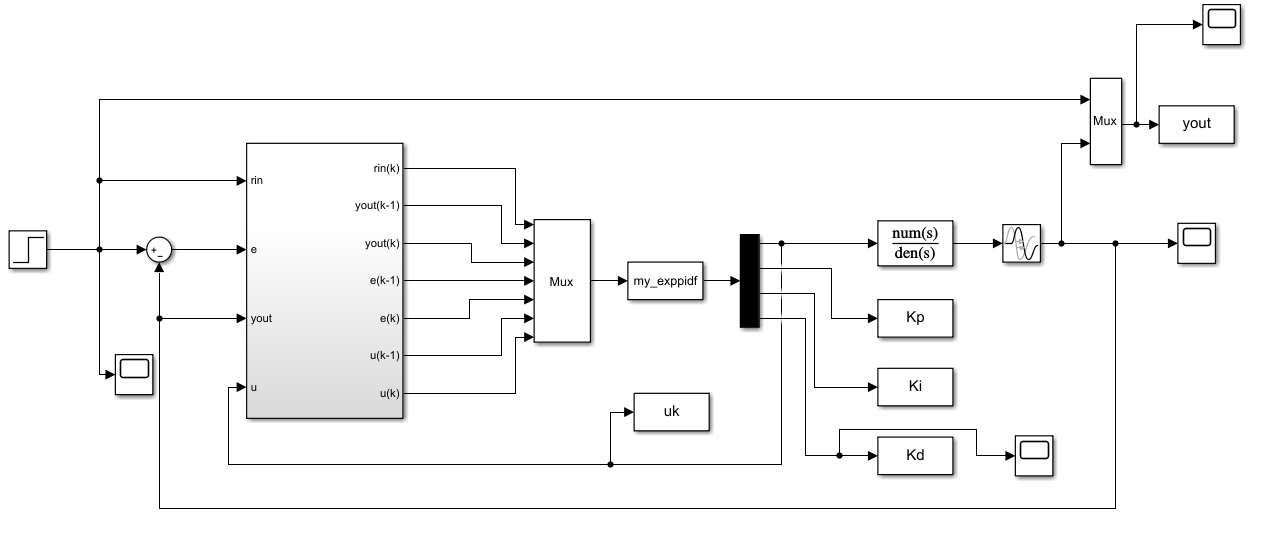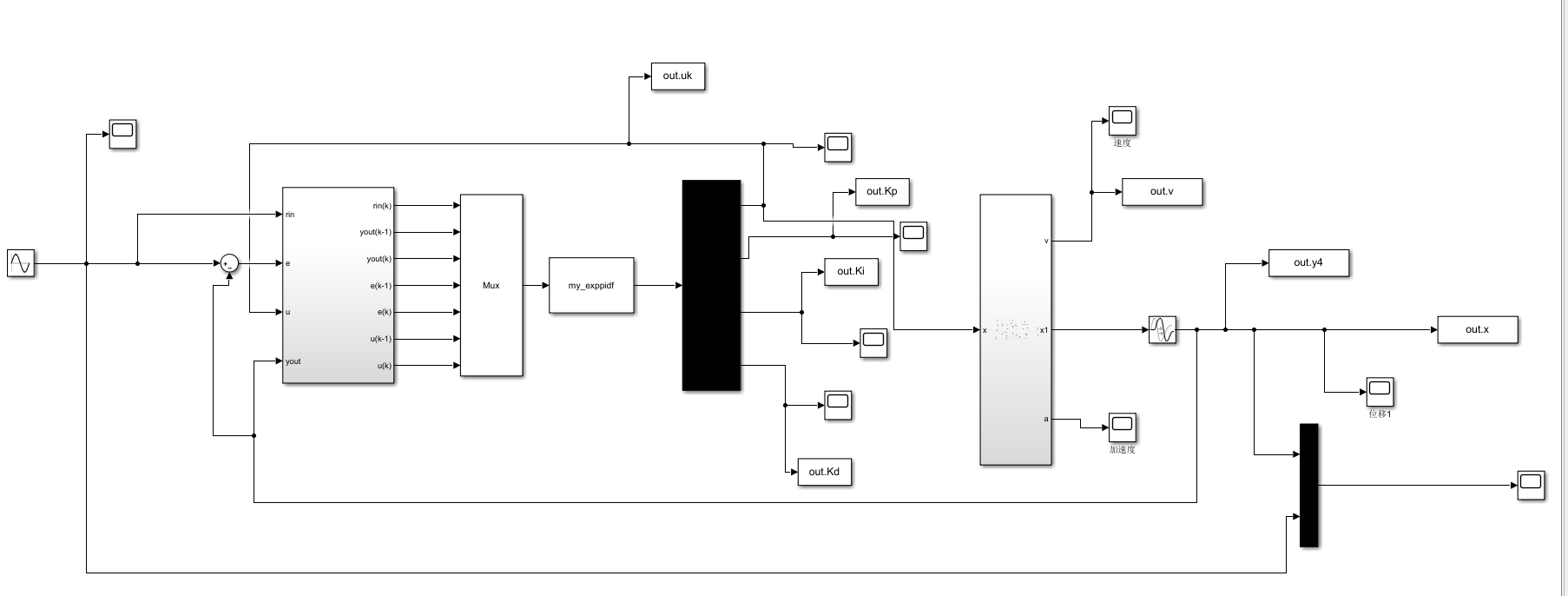function [sys,x0,str,ts]=my_exppidf(t,x,u,flag)
switch flag,
case 0,
[sys,x0,str,ts]=mdlInitializeSizes;
case 2,
case 3,
sys=mdlOutputs(t,x,u);
case {1,4,9},
sys=[];
otherwise
error(['unhandled flag=',num2str(flag)]);%异常处理
end
function[sys,x0,str,ts]=mdlInitializeSizes
sizes=simsizes;%用于设置模块参数的结构体用simsizes来生成
sizes.NumContStates=0;%模块连续状态变量的个数
sizes.NumDiscStates=3;%模块离散状态变量的个数
sizes.NumOutputs=4;%模块输出变量的个数
sizes.NumInputs=7;%模块输入变量的个数
sizes.DirFeedthrough=1;%模块是否存在直接贯通，1表示存在直接贯通，若为0，则mdlOutputs函数里不能有u
sizes.NumSampleTimes=1;%模块的采样时间个数,至少是一个
sys=simsizes(sizes);%设置完后赋给sys输出
x0=zeros(3,1);%系统状态变量设置
str=[];
ts=[0 0];%采样周期设为0表示是连续系统，
%     ts=[0.001 0];%采样周期设为0表示是连续系统，
T=0.001;
x=[u(5);x(2)+u(5)*T;(u(5)-u(4))/T];%3个状态量（偏差、偏差和以及偏差变化量），u(5)是偏差，u(4)是上一次的偏差，x(2)则是之前的偏差和
sys=[x(1);x(2);x(3)];
function sys=mdlOutputs(t,x,u)
xite=0.2;
alfa=0.05;
IN=3;H=5;OUT=3;
wi=rand(5,3);%产生一个5*3的随机数矩阵，随机数在（0，1）区间
wi_1=wi;wi_2=wi;wi_3=wi;
wo=rand(3,5);
wo_1=wo;wo_2=wo;wo_3=wo;
Oh=zeros(5,1);%产生一个1*5的零矩阵(行矩阵)
I=Oh;
xi=[u(1),u(3),u(5)];%神经网络训练的3个输入，期望值、误差以及实际值
epid=[x(1);x(2);x(3)];%3个状态变量（偏差、偏差和、偏差变化量）（3*1矩阵，列向量）
I=xi*wi';%隐层的输入
for j=1:1:5
Oh(j)=(exp(I(j))-exp(-I(j)))/(exp(I(j))+exp(-I(j)));%隐层的输出值（1*5矩阵）行矩阵
end
K1=wo*Oh;%输出层的输入（3*1矩阵）
for i=1:1:3
K(i)=exp(K1(i))/(exp(K1(i))+exp(-K1(i)));%得到输出层的输出（KP、KI、KD）（1*3矩阵，行向量）
end
u_k=K*epid;%计算得到控制律u，1个值
%%以下是权值调整
%隐含层至输出层的权值调整
dyu=sign((u(3)-u(2))/(u(7)-u(6)+0.0001));
for j=1:1:3
dK(j)=2/(exp(K1(j))+exp(-K1(j)))^2; %输出层的输出的一阶导
end
for i=1:1:3
delta3(i)=u(5)*dyu*epid(i)*dK(i);  %输出层的delta
end
for j=1:1:3
for i=1:1:5
d_wo=xite*delta3(j)*Oh(i)+alfa*(wo_1-wo_2);
end
end
wo=wo_1+d_wo;
%以下是输入层至隐含层的权值调整
for i=1:1:5
dO(i)=4/(exp(I(i))+exp(-I(i)))^2;%(1*5矩阵)
end
segma=delta3*wo;%（1*5矩阵，行向量）
delta2 = dO.*segma;
d_wi = delta2'*xi+alfa*(wi_1-wi_2);
wi=wi_1+d_wi;
wo_3=wo_2;
wo_2=wo_1;
wo_1=wo;%储存输出层本次调整后的权值
wi_3=wi_2;
wi_2=wi_1;
wi_1=wi;%储存隐层本次调整后的权值
Kp=K(1);Ki=K(2);Kd=K(3);
sys=[u_k,Kp,Ki,Kd];       

1、仿真设置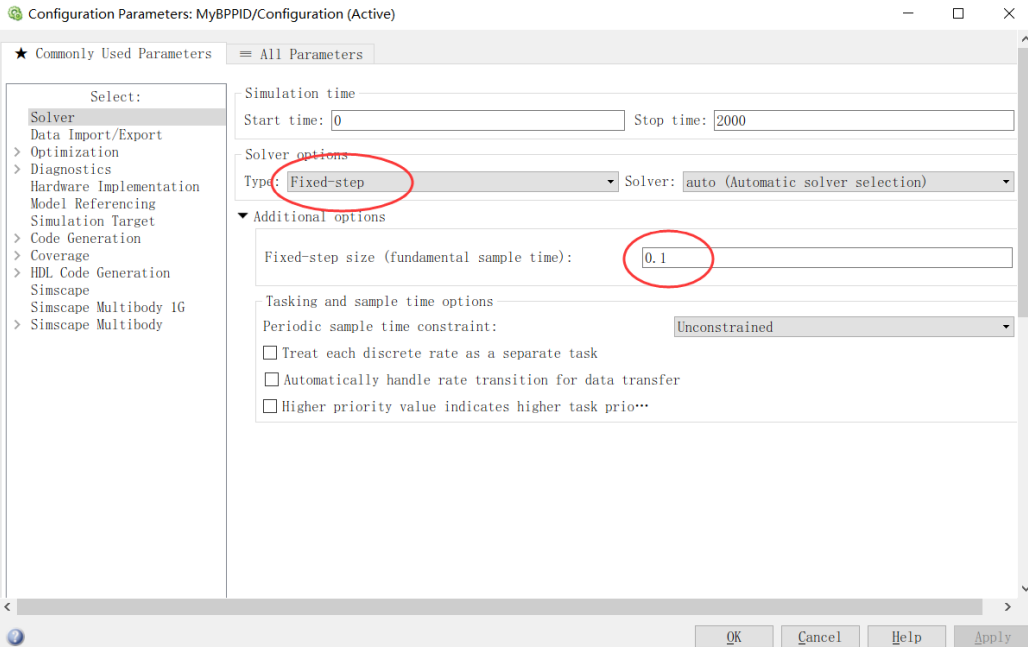2、关于 Transport Delay ，文章中要求延时80,但本例子中设置不延时，如果延时80s会出现一直是0直线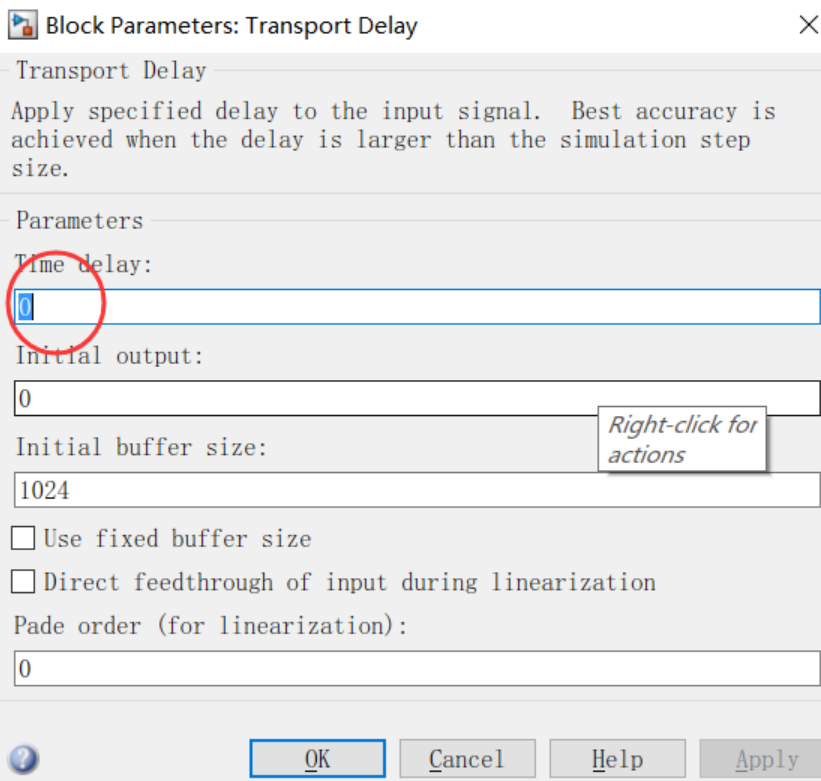展开全文simulink仿真
• BP神经网络PID控制，可以对预定数据进行快速跟踪，误差较小，路径可以根据自己需要设定。

万次阅读 多人点赞 2019-05-30 10:58:30
最近在学习电机的智能控制，上周学习了基于单神经元的PID控制，这周研究基于BP神经网络PID控制。 神经网络具有任意非线性表达能力，可以通过对系统性能的学习来实现具有最佳组合的PID控制。利用BP神经网络可以...

最近在学习电机的智能控制，上周学习了基于单神经元的PID控制，这周研究基于BP神经网络的PID控制。

神经网络具有任意非线性表达能力，可以通过对系统性能的学习来实现具有最佳组合的PID控制。利用BP神经网络可以建立参数Kp,Ki,Kd自整定的PID控制器。基于BP神经网络的PID控制系统结构框图如下图所示：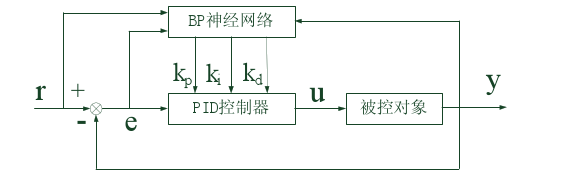控制器由两部分组成：经典增量式PID控制器；BP神经网络

经典增量式PID控制器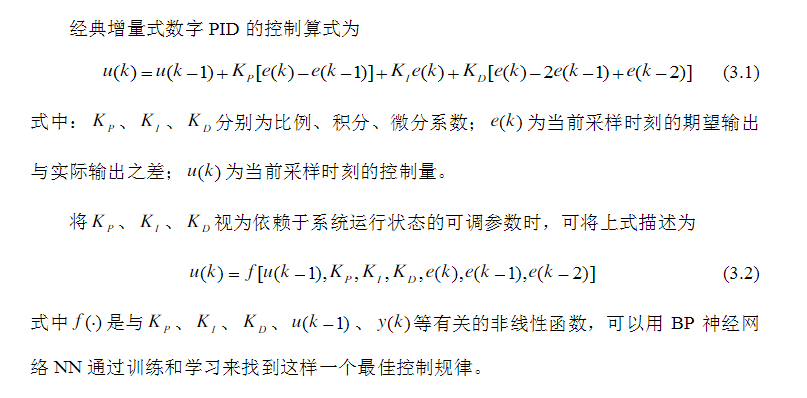BP神经网络控制算法

BP神经网络结构如下图所示：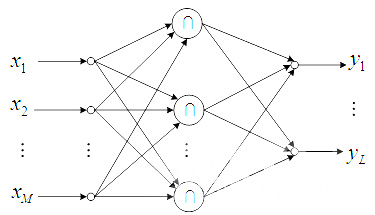它是一种有隐含层的3层前馈网络，包括输入层、隐含层和输出层。输出层的三个输出分别对应PID控制器的三个可调参数Kp、Ki和Kd。由于Kp、Ki和Kd不能为负，所以输出层神经元的变换函数取非负的Sigmoid函数，而隐含层神经元的变换函数可取正负对称的Sigmoid函数。

BP神经网络的输入（M为输入变量的个数）：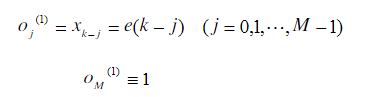隐含层的输入输出为：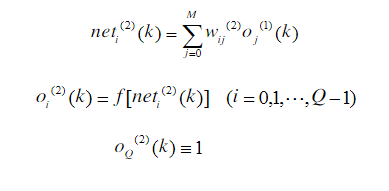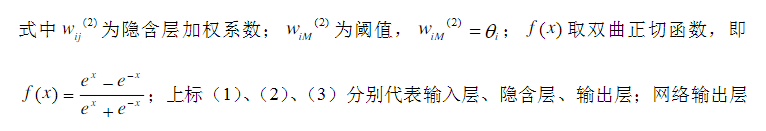输出层的输入输出：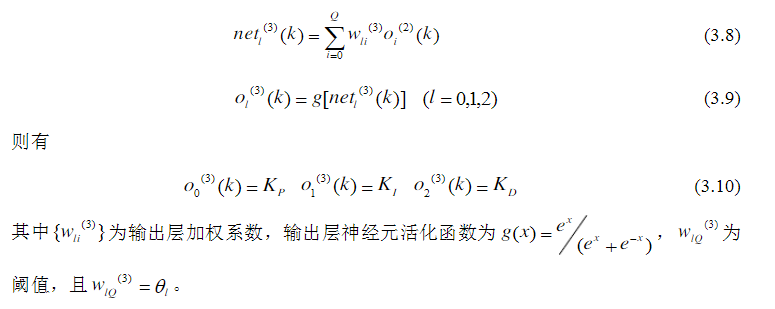采用以输出误差二次方为性能指标，其性能指标函数为：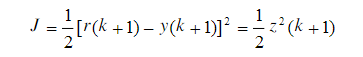按照梯度下降法修正网络的加权系数，并附加一使搜索快速收敛全局极小的惯性项，则有BP神经网络输出层的加权系数修正公式为：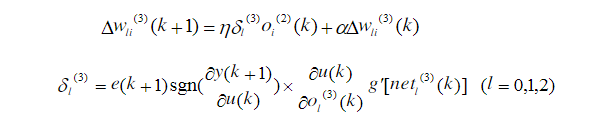同理，可得隐含层加权系数的计算公式为：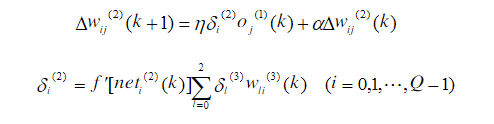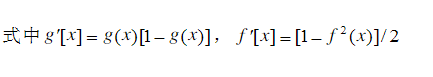由此，BP神经网络PID控制算法可总结为：

（1）确定BP神经网络结构，即确定输入层和隐含层的节点个数，选取各层加权系数的初值wij(0)、wli(0),选定学习速率和惯性系数，此时k=1

（2）采样给定和反馈信号，即r(k)和y(k),计算误差e(k)=r(k)-y(k)

（3）确定输入量

（4）根据上述公式，计算各层神经元的输入、输出，神经网络输出层即为PID控制器的三个可调参数Kp、Ki和Kd

（5）由增量式PID控制公式，计算PID控制器的控制输出u(k)

（6）进行神经网络学习，实时自动调整输出层和隐含层的加权系数wli(k)和wij(k)，实现PID控制参数的自适应调整

（7）置k=k+1，返回步骤（2）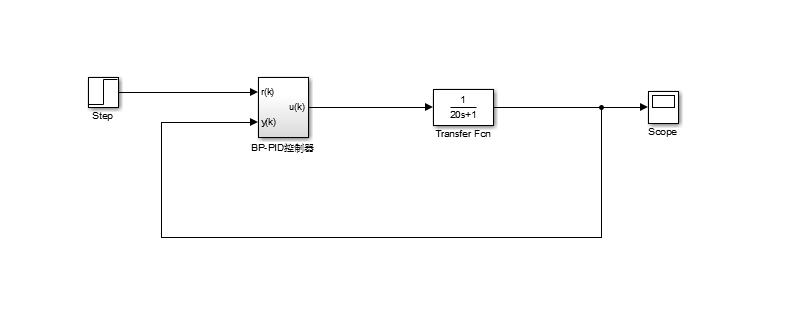输入为阶跃信号，其参数为默认值，一个简单的闭环控制系统。BP神经网络PID控制器的内部结构如下图所示：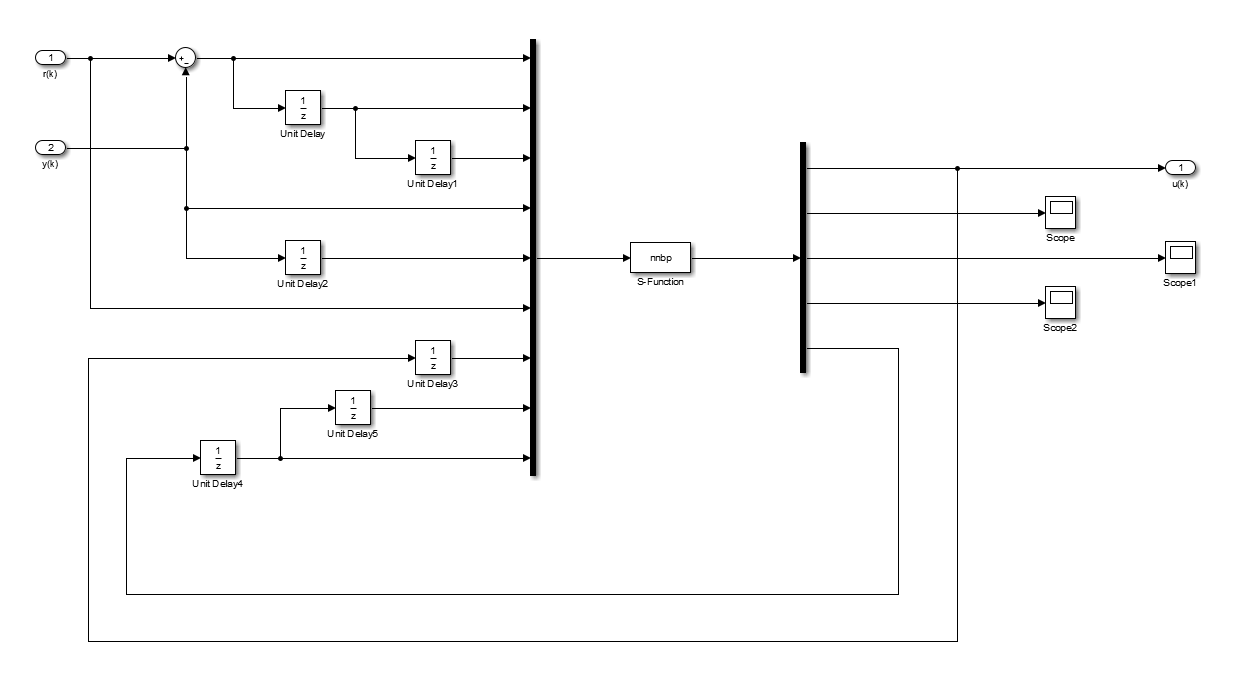S-function的输入为:u=[e(k);e(k-1);e(k-2);y(k);y(k-1);r(k);u(k-1);隐含层+输出层权值系数(k-2);隐含层+输出层权值系数(k-1)]=

[u(1);u(2);u(3);u(4);u(5);u(6);u(7);...u(隐含层权值个数+输出层权值个数）]，把所有的权值系数从输出再返回到输入是为了更新权值矩阵，从而自适应的调整PID三个参数。关于S-function的使用方法，请参考我写的另一篇博客：           https://blog.csdn.net/weixin_42650162/article/details/90488610

S-function函数

下面是S-function函数编写的控制算法：

为了更好的理解下面的程序代码，先要理解Matlab中的几个函数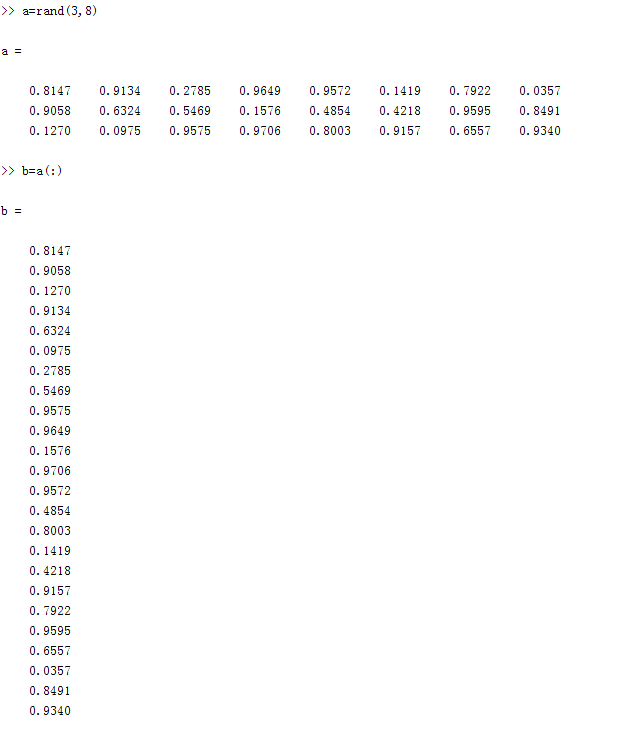通过(:)把一个矩阵变为一个列向量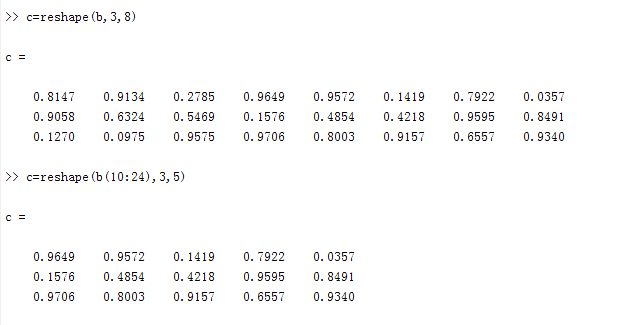通过reshape函数，从列向量里任意组成矩阵如c=reshape(b,3,8)，b中元素按顺序排成一个3*8的矩阵，也就是还原了矩阵a，

c=reshape(b(10:24),3,5)，b中第10个元素到第24个元素，按顺序排成一个3*5的矩阵。

在我编写的S-function函数中，就是通过reshape函数，把输入的隐含层+输出层的列权值系数还原成：隐含层权值系数矩阵+输出层权值系数矩阵，通过算法完成这两个权值系数矩阵的更新。

下面是M文件编写的S-function控制算法：

function [sys,x0,str,ts,simStateCompliance] = nnbp(t,x,u,flag,T,nh,xite,alfa)
switch flag,
case 0,
[sys,x0,str,ts,simStateCompliance]=mdlInitializeSizes(T,nh);
%初始化函数
case 3,
sys=mdlOutputs(t,x,u,nh,xite,alfa);
%输出函数
case {1,2,4,9},
sys=[];
otherwise
end
function [sys,x0,str,ts,simStateCompliance]=mdlInitializeSizes(T,nh)
%调用初始画函数，两个外部输入参数 参数T确定采样时间，参数nh确定隐含层层数
sizes = simsizes;
sizes.NumContStates  = 0;
sizes.NumDiscStates  = 0;
sizes.NumOutputs     = 4+6*nh;
%定义输出变量，包括控制变量u,三个PID参数：Kp,Ki,Kd,隐含层+输出层所有加权系数
sizes.NumInputs      = 7+12*nh;
%定义输入变量，包括前7个参数[e(k);e(k-1);e(k-2);y(k);y(k-1);r(k);u(k-1)]
%隐含层+输出层权值系数（k-2),隐含层+输出层权值系数（k-1）
sizes.DirFeedthrough = 1;
sizes.NumSampleTimes = 1;
sys = simsizes(sizes);
x0  = [];
str = [];
ts  = [T 0];
simStateCompliance = 'UnknownSimState';
function sys=mdlOutputs(t,x,u,nh,xite,alfa)
%调用输出函数
wi_2 = reshape(u(8:7+3*nh),nh,3);
%隐含层（k-2)权值系数矩阵，维数nh*3
wo_2 = reshape(u(8+3*nh:7+6*nh),3,nh);
%输出层（k-2）权值系数矩阵，维数3*nh
wi_1 = reshape(u(8+6*nh:7+9*nh),nh,3);
%隐含层（k-1)权值系数矩阵，维数nh*3
wo_1 = reshape(u(8+9*nh:7+12*nh),3,nh);
%输出层（k-1）权值系数矩阵，维数3*nh
xi = [u(6),u(4),u(1)];
%神经网络的输入xi=[u(6),u(4),u(1)]=[r(k),y(k),e(k)]
xx = [u(1)-u(2);u(1);u(1)+u(3)-2*u(2)];
%xx=[u(1)-u(2);u(1);u(1)+u(3)-2*u(2)]=[e(k)-e(k-1);e(k);e(k)+e(k-2)-2*e(k-1)]
I = xi*wi_1';
%计算隐含层的输入，I=神经网络的输入*隐含层权值系数矩阵的转置wi_1'，结果为：
%I=[net0(k),net1(k)...netnh(k)]为1*nh矩阵
Oh = exp(I)./(exp(I)+exp(-I));
%激活函数，可更改
%计算隐含层的输出，(exp(I)-exp(-I))./(exp(I)+exp(-I))为隐含层的激活函数Sigmoid
%Oh=[o0(k),o1(k)...onh(k)],为1*nh的矩阵
O = wo_1*Oh';
%计算输出层的输入，维数3*1
K = 2./(exp(O)+exp(-O)).^2;
%激活函数，可更改
%计算输出层的输出K=[Kp,Ki,Kd]，维数为1*3
%exp(Oh)./(exp(Oh)+exp(-Oh))为输出层的激活函数Sigmoid
uu = u(7)+K'*xx;
%根据增量式PID控制算法计算控制变量u(k)
dyu = sign((u(4)-u(5))/(uu-u(7)+0.0000001));
%计算输出层加权系数修正公式的sgn
%sign((y(k)-y(k-1))/(u(k)-u(k-1)+0.0000001)近似代表偏导
dK = 2./(exp(K)+exp(-K)).^2;
%激活函数，可更改
delta3 = u(1)*dyu*xx.*dK;
wo = wo_1+xite*delta3*Oh+alfa*(wo_1-wo_2);
%输出层加权系数矩阵的修正
dOh = 2./(exp(Oh)+exp(-Oh)).^2;
%激活函数，可更改
wi = wi_1+xite*(dOh.*(delta3'*wo))'*xi+alfa*(wi_1-wi_2);
%隐含层加权系数修正
sys = [uu;K(:);wi(:);wo(:)];
%输出层输出sys=[uu;K(:);wi(:);wo(:)]=
%[uu;Kp;Ki;Kd;隐含层+输出层所有权值系数]
%K(:),wi(:),wo(:),把这三个矩阵按顺序排为列向量

本函数有四个外部输入变量：T，nh，xite,alfa T输入采样时间，nh确定隐含层层数，xite和alfa权值系数修正公式里的学习速率和惯性系数。

为了更好地分配S-function的输出，需要对Demux进行如下设置：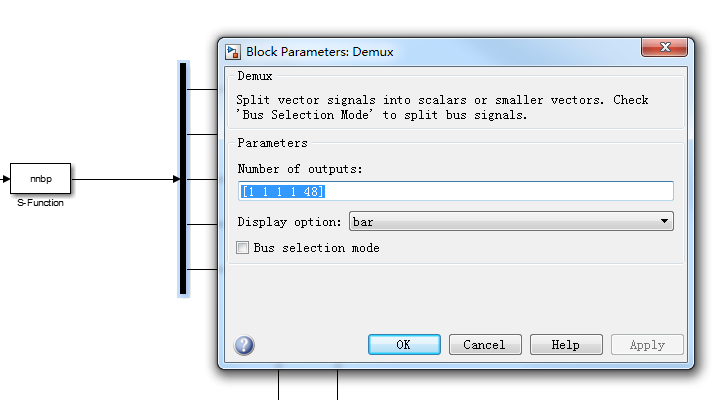确保前三个输出变量为：控制变量u,Kp,Ki,Kd,剩下的变量为隐含层权值系数矩阵+输出层权值系数矩阵总数之和。

然后对此S-function函数进行封装：

具体过程可以参考我的另一篇博客：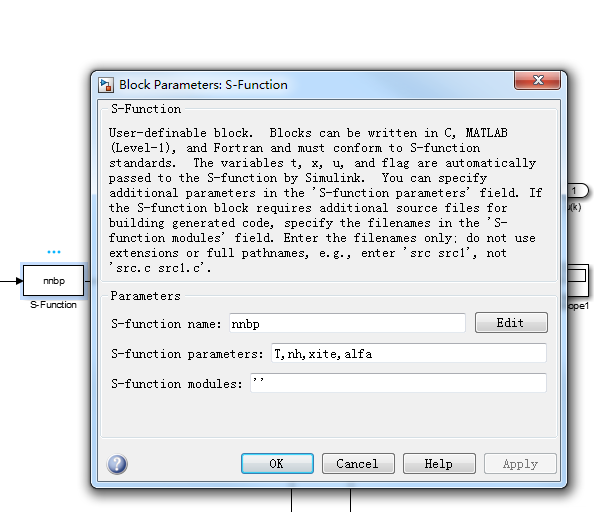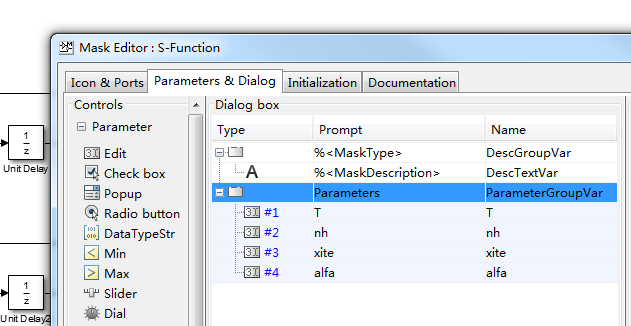完成后点击S-function函数，会弹出外部参数设置框，设置参数如下：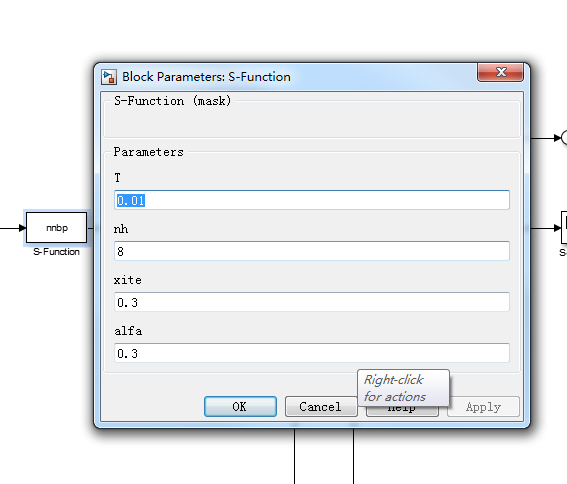注：T采样时间要和设置的控制算法的采样时间一样，不然会报错，如下：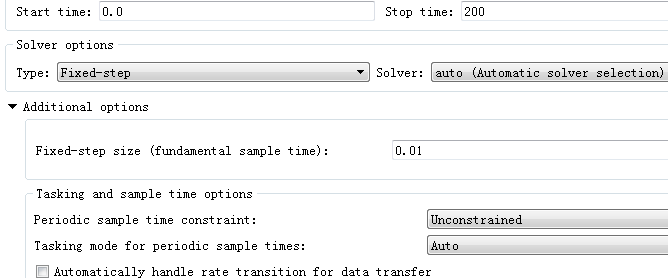下面是仿真结果：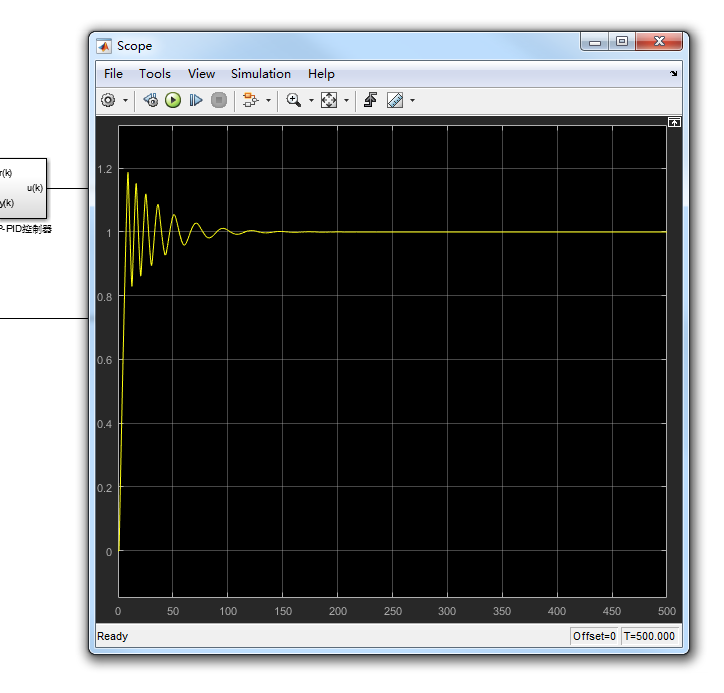Kp、Ki、Kd的自适应变化曲线：

Kp：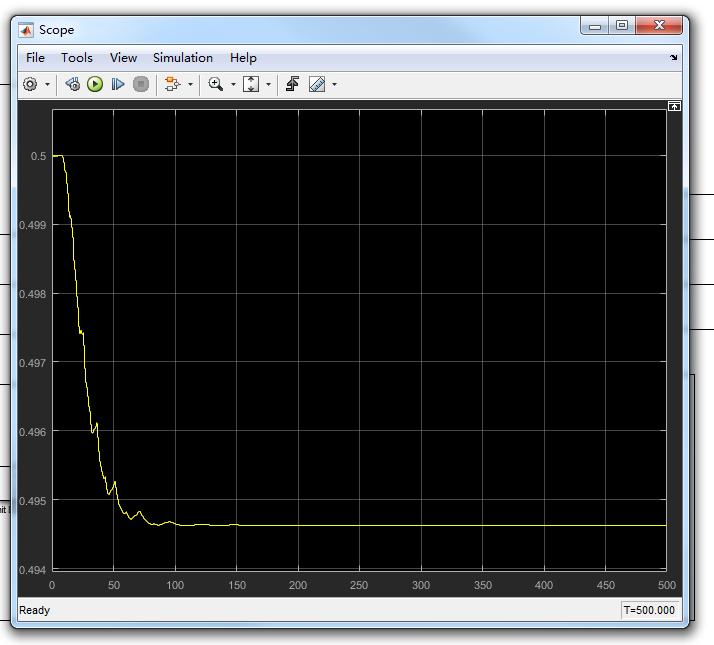Ki：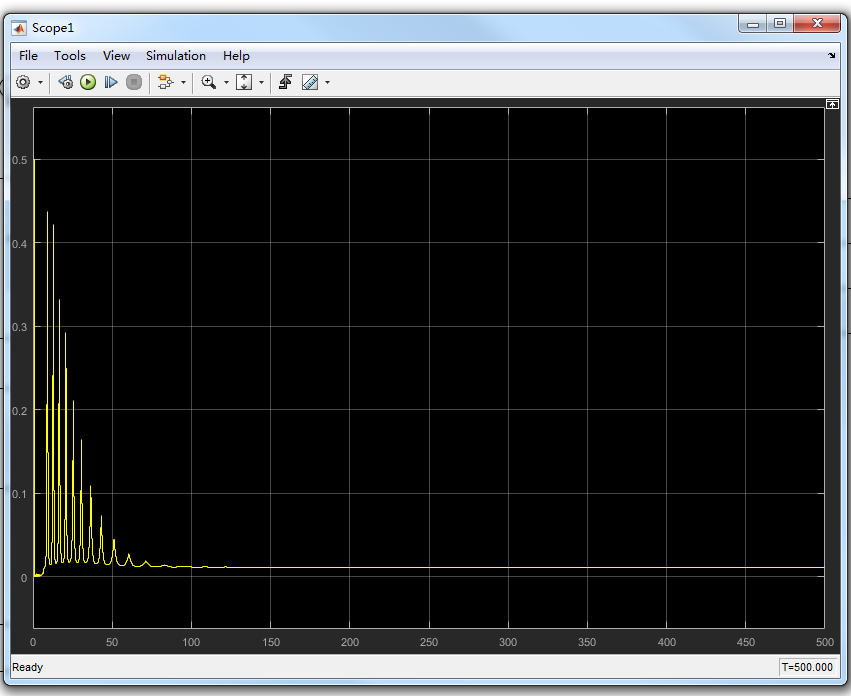Kd: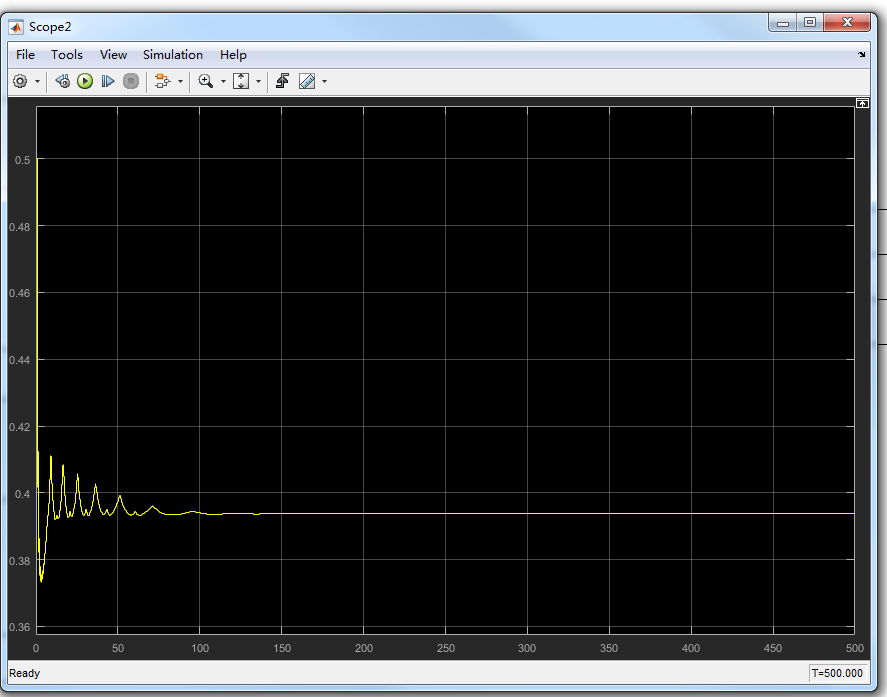有关仿真的所有资源已上传，如有需要可自行下载：

展开全文Simulink仿真
• 基于MATLAB的BP神经网络PID控制器设计 一、BP神经网络PID控制算法步骤： （1）确定BP神经网络结构，即确定输入层和隐含层的节点个数，选取各层加权系数的初值wij(0)、wli(0),选定学习速率和惯性系数，此时k=1 （2）...

## 基于MATLAB的BP神经网络PID控制器设计

### 一、BP神经网络PID控制算法步骤：

（1）确定BP神经网络结构，即确定输入层和隐含层的节点个数，选取各层加权系数的初值wij(0)、wli(0),选定学习速率和惯性系数，此时k=1

（2）采样给定和反馈信号，即r(k)和y(k),计算误差e(k)=r(k)-y(k)

（3）确定输入量

（4）根据上述公式，计算各层神经元的输入、输出，神经网络输出层即为PID控制器的三个可调参数Kp、Ki和Kd

（5）由增量式PID控制公式，计算PID控制器的控制输出u(k)

（6）进行神经网络学习，实时自动调整输出层和隐含层的加权系数wli(k)和wij(k)，实现PID控制参数的自适应调整

（7）置k=k+1，返回步骤（2）


### 二、建立模型

##### 2.1 BP_PID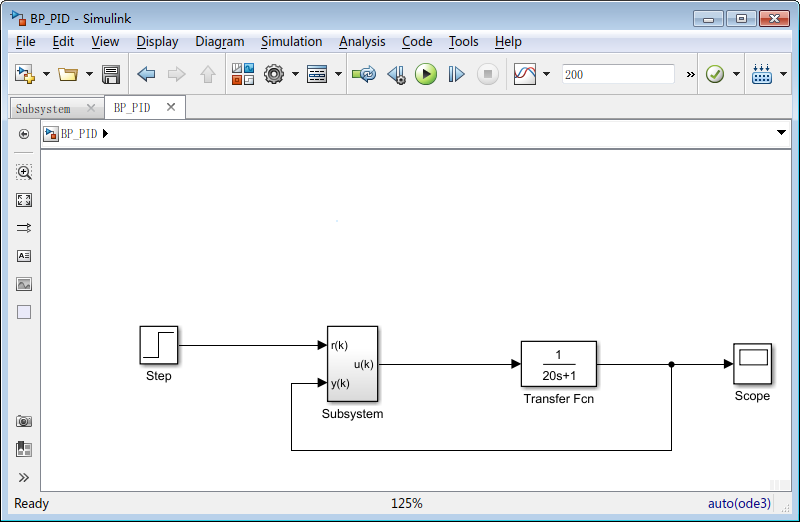##### 2.2 Subsystem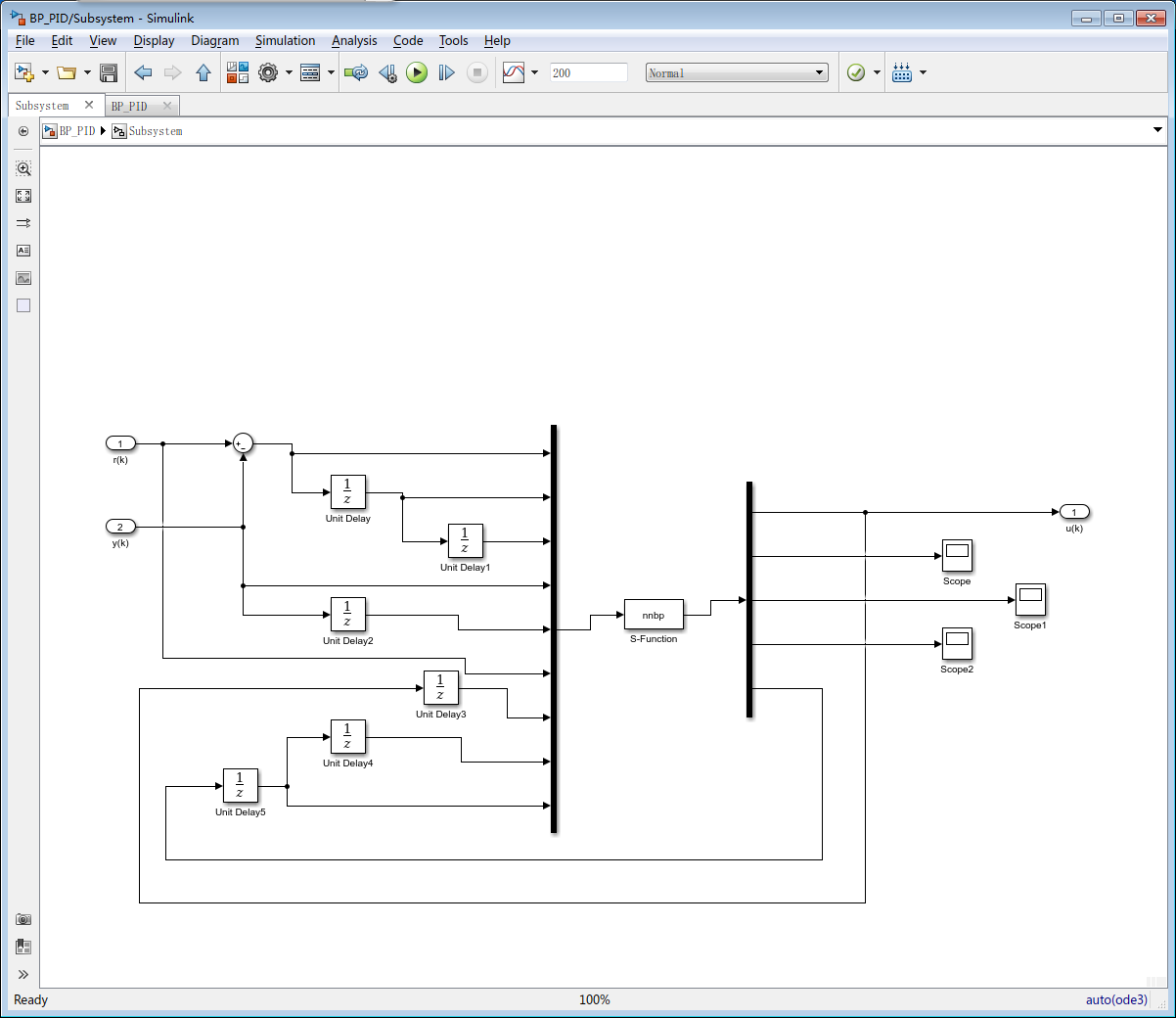##### 2.3 S_Function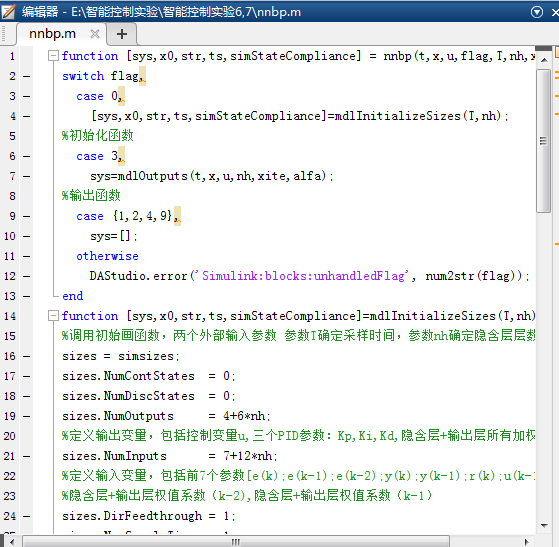function [sys,x0,str,ts,simStateCompliance] = nnbp(t,x,u,flag,T,nh,xite,alfa)
switch flag,
case 0,
[sys,x0,str,ts,simStateCompliance]=mdlInitializeSizes(T,nh);
%初始化函数
case 3,
sys=mdlOutputs(t,x,u,nh,xite,alfa);
%输出函数
case {1,2,4,9},
sys=[];
otherwise
end
function [sys,x0,str,ts,simStateCompliance]=mdlInitializeSizes(T,nh)
%调用初始画函数，两个外部输入参数 参数T确定采样时间，参数nh确定隐含层层数
sizes = simsizes;
sizes.NumContStates  = 0;
sizes.NumDiscStates  = 0;
sizes.NumOutputs     = 4+6*nh;
%定义输出变量，包括控制变量u,三个PID参数：Kp,Ki,Kd,隐含层+输出层所有加权系数
sizes.NumInputs      = 7+12*nh;
%定义输入变量，包括前7个参数[e(k);e(k-1);e(k-2);y(k);y(k-1);r(k);u(k-1)]
%隐含层+输出层权值系数（k-2),隐含层+输出层权值系数（k-1）
sizes.DirFeedthrough = 1;
sizes.NumSampleTimes = 1;
sys = simsizes(sizes);
x0  = [];
str = [];
ts  = [T 0];
simStateCompliance = 'UnknownSimState';
function sys=mdlOutputs(t,x,u,nh,xite,alfa)
%调用输出函数
wi_2 = reshape(u(8:7+3*nh),nh,3);
%隐含层（k-2)权值系数矩阵，维数nh*3
wo_2 = reshape(u(8+3*nh:7+6*nh),3,nh);
%输出层（k-2）权值系数矩阵，维数3*nh
wi_1 = reshape(u(8+6*nh:7+9*nh),nh,3);
%隐含层（k-1)权值系数矩阵，维数nh*3
wo_1 = reshape(u(8+9*nh:7+12*nh),3,nh);
%输出层（k-1）权值系数矩阵，维数3*nh
xi = [u(6),u(4),u(1)];
%神经网络的输入xi=[u(6),u(4),u(1)]=[r(k),y(k),e(k)]
xx = [u(1)-u(2);u(1);u(1)+u(3)-2*u(2)];
%xx=[u(1)-u(2);u(1);u(1)+u(3)-2*u(2)]=[e(k)-e(k-1);e(k);e(k)+e(k-2)-2*e(k-1)]
I = xi*wi_1';
%计算隐含层的输入，I=神经网络的输入*隐含层权值系数矩阵的转置wi_1'，结果为：
%I=[net0(k),net1(k)...netnh(k)]为1*nh矩阵
Oh = exp(I)./(exp(I)+exp(-I));
%激活函数，可更改
%计算隐含层的输出，(exp(I)-exp(-I))./(exp(I)+exp(-I))为隐含层的激活函数Sigmoid
%Oh=[o0(k),o1(k)...onh(k)],为1*nh的矩阵
O = wo_1*Oh';
%计算输出层的输入，维数3*1
K = 2./(exp(O)+exp(-O)).^2;
%激活函数，可更改
%计算输出层的输出K=[Kp,Ki,Kd]，维数为1*3
%exp(Oh)./(exp(Oh)+exp(-Oh))为输出层的激活函数Sigmoid
uu = u(7)+K'*xx;
%根据增量式PID控制算法计算控制变量u(k)
dyu = sign((u(4)-u(5))/(uu-u(7)+0.0000001));
%计算输出层加权系数修正公式的sgn
%sign((y(k)-y(k-1))/(u(k)-u(k-1)+0.0000001)近似代表偏导
dK = 2./(exp(K)+exp(-K)).^2;
%激活函数，可更改
delta3 = u(1)*dyu*xx.*dK;
wo = wo_1+xite*delta3*Oh+alfa*(wo_1-wo_2);
%输出层加权系数矩阵的修正
dOh = 2./(exp(Oh)+exp(-Oh)).^2;
%激活函数，可更改
wi = wi_1+xite*(dOh.*(delta3'*wo))'*xi+alfa*(wi_1-wi_2);
%隐含层加权系数修正
sys = [uu;K(:);wi(:);wo(:)];
%输出层输出sys=[uu;K(:);wi(:);wo(:)]=
%[uu;Kp;Ki;Kd;隐含层+输出层所有权值系数]
%K(:),wi(:),wo(:),把这三个矩阵按顺序排为列向量



### 三、实验结果及分析

##### 3.1 output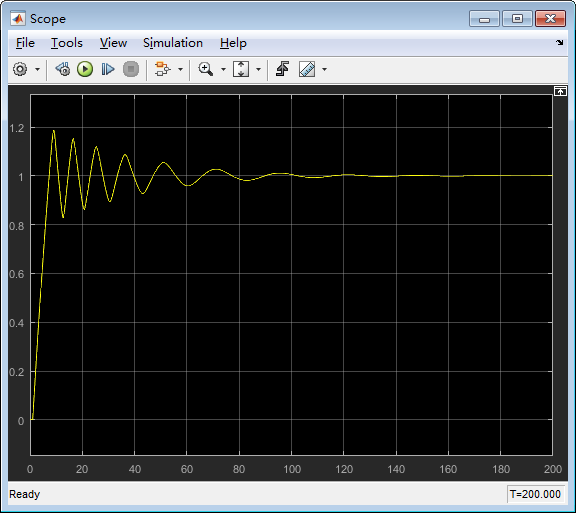##### 3.2 kp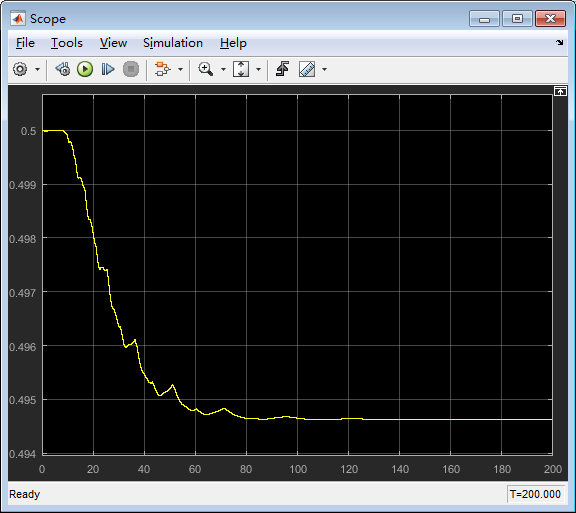##### 3.3 ki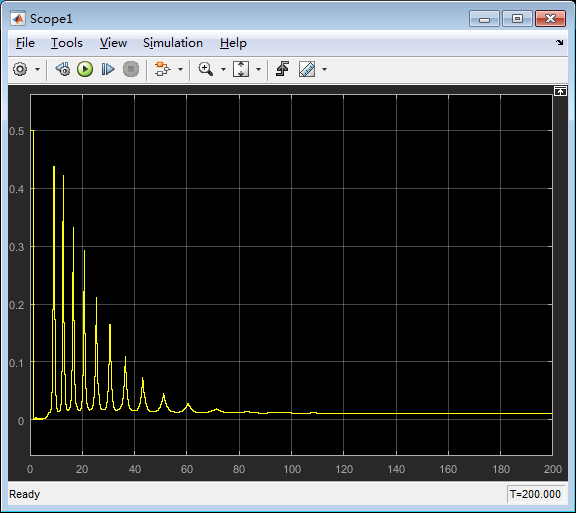##### 3.4 kd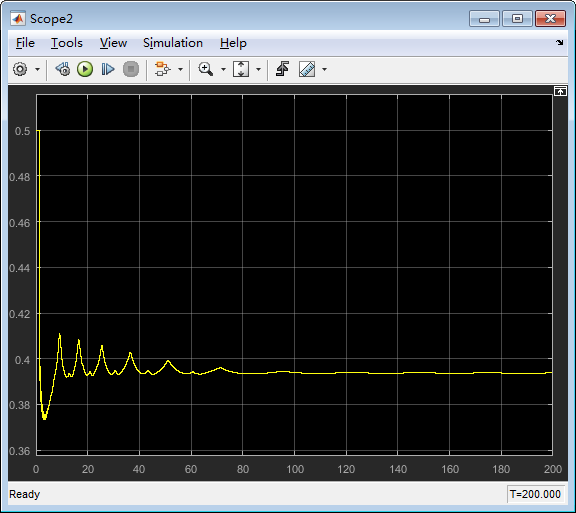### 参考文章

展开全文matlab 开发语言
• Matlab基于BPPID神经网络控制-基于BP PID神经网络控制.rar 基于BP PID神经网络控制，不错的！！！matlab
• 基于PSO优化BP神经网络PID控制器的仿真,使用matlab2021a或者更高版本测试 for j=1:1:H Oh(j)=( exp( net2(j)-exp(-net2(j)) ) )/(exp( net2(j)+exp(-net2(j)) )); end net3=wo*Oh; for l=1:1:Out K(l)=exp...matlab
• 基于BP神经网络PID控制的方法研究，孙广孟，，PID控制是一种经典的控制方法，它以结构简单，不依赖于被控对象的数学模型，抗干扰能力强等优点，成为了过程控制领域一种重要的方
• BP PID控制器，加入了一个传递函数作为案例，能够实现优化PID算法，
• %BP based PID Controlclear all;close all;xite=0.25;alfa=0.05;S=2; %Signal typeIN=4;H=5;Out=3; %NN Structureif S==1 %Step Signalwi=[-0.6394 -0.2696 -0.3756 -0.7023;-0.8603 -0.2013 -0.5024 -0.2596;-1.07...
• 内容介绍原文档由会员 hk10101 发布利用BP神经网络PID控制器进行优化PID控制是最早发展起来的控制策略之一，由于其算法简单、鲁棒性好和可靠性高，被广泛应用于工业控制过程，尤其适用于可建立精确数学模型的确定性...
• 考虑MPSO的变异机制，以种群适应度方差与种群最优适应度值为标准，进行种群变异操作，可以克服早熟，提高收敛精度和PSO的全局搜索能力，使MPSO优化的BP神经网络整定的PID控制器能以更快的速度、更高的精度完成过程...
• 学 海 无 涯 MATLAB 基于BP 神经网络PID 控制程序 > %BP based PID Control clear all; close all; xite=0.20; %学习速率 alfa=0.01; %惯性因子 IN=4;H=5;Out=3; %NN Structure wi=[-0.6394 -0.2696 -0.3756 -0.7023...
• BP神经网络优化PID程序,bp神经网络pid控制,C,C++源码
• BP网络PID控制器,bp神经网络pid控制,matlab源码...